# Ratio of sides 2

The ratio of the side lengths of one square to another is 1:2. Find the ratio of the area of the two squares.

Result

r =  1:4

#### Solution:Leave us a comment of example and its solution (i.e. if it is still somewhat unclear...):

Showing 0 comments:Be the first to comment!## Next similar examples:

1. DonutsFind how many donuts each student will receive if you share 126 donuts in a ratio of 1:5:8
2. Two numbersFind two numbers whose difference and ratio is 2.
3. Land areaA land area of Asia and Africa are in a 3: 2 ratio, the European and African are is 1:3. What are the proportions of Asia, Africa, and Europe?
4. In theIn the national park, the ratio of the wooded area to grassland is 4: 1. The total area is 385km2. What area is wooded?
5. AgesJohn, Teresa, Daniel and Paul have summary 56 years. Their ages are in a ratio of 1:2:5:6. Determine how many years have each of them.
6. PeppersIn the box are yellow (a), green (b) and red (c) peppers. Their amount is in a ratio 2:4:1 . Most are yellow peppers and green the least. Calculate the number of peppers each type if the total number of peppers is 70.
7. Image scale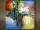The actual image dimensions are 60 cm x 80cm and has a reduced size 3 cm x 4 cm. At what scale the image was reduced?
8. Divide 5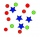Divide 288 in the following ratio 3 : 4 : 5
9. Line segmentThe 4 cm long line segment is enlarged in the ratio of 5/2. How many centimeters will measure the new line segment?
10. Arble bagA marble bag sold by Rachel's Marble Company contains 5 orange marbles for every 6 green marbles. If a bag has 35 orange marbles, how many green marbles does it contain?
11. 1.5 divided1.5 divided by 1 = w divided by 4
12. RunnersFor three runners (on the first to third place) is prepared 30 chocolate that they be distributed in the ratio of 3 : 2 : 1 How much chocolate will get everyone?
13. Euros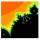Michal, Peter, John and Lenka got together 2,400 euros. They share an amount in ratio 2:6:4:3. How many got each of them?
14. Areaf of ST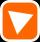It is given square DBLK with side |BL|=13. Calculate area of triangle DKU if vertex U lie on line LB.
15. Trapezium 2Trapezium has an area of 24 square cms. How many different trapeziums can be formed ?
16. Masons1 mason casts 30.8 meters square in 8 hours. How long casts 4 masons 178 meters square?
17. Percent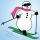From 99 children who participated in the ski course were 23 excellent skiers, 13 good and 20 average and the rest were beginners. Calculate this data in percentages.